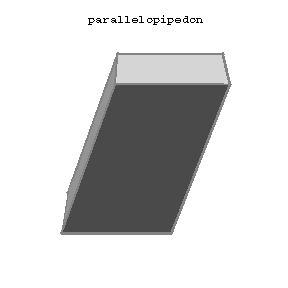# Difference between revisions of "Parallelopipedon"

A hexahedron whose opposite faces are pairwise parallel. A parallelopipedon has 8 vertices and 12 edges; its faces are pairwise congruent parallelograms. A parallelopipedon is called rectangular if the 6 faces are rectangles; a parallelopipedon all faces of which are squares is called a cube. The volume of a parallelopipedon is equal to the product of the area of its base and its height.Figure: p071450a

A parallelopipedon is a special case of a parallelohedron and of a parallelotope. Two special parallelopipeda, namely the golden rhombohedra or Ammann rhombohedra, play an essential role in the theory of quasi-crystals, because they are the $3$-dimensional analogues of the Penrose tiles: They generate aperiodic tilings of $\mathbf R^3$.